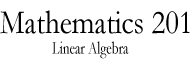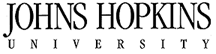Math 201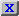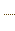Homework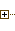Past ExamsQuizzes & SolutionsSyllabusStatement on Ethics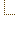Supportive material
 Math Dept. Home Help room

Quizzes-Midterms-Final

Here you can find the test and the solution of the assigned biweekly quizzes

[27 students performed *<= 40/100; 42 students performed 41/100<= * <= 55/100; 23 students performed 56/100 <= * <= 65/100; 17 students performed 66/100 <= * <= 80/100; 7 students performed * >= 81/100]

[18 students performed *<= 40/100; 25 students performed 41/100<= * <= 55/100; 27 students performed 56/100 <= * <= 65/100; 37 students performed 66/100 <= * <= 80/100; 5 students performed * >= 81/100]